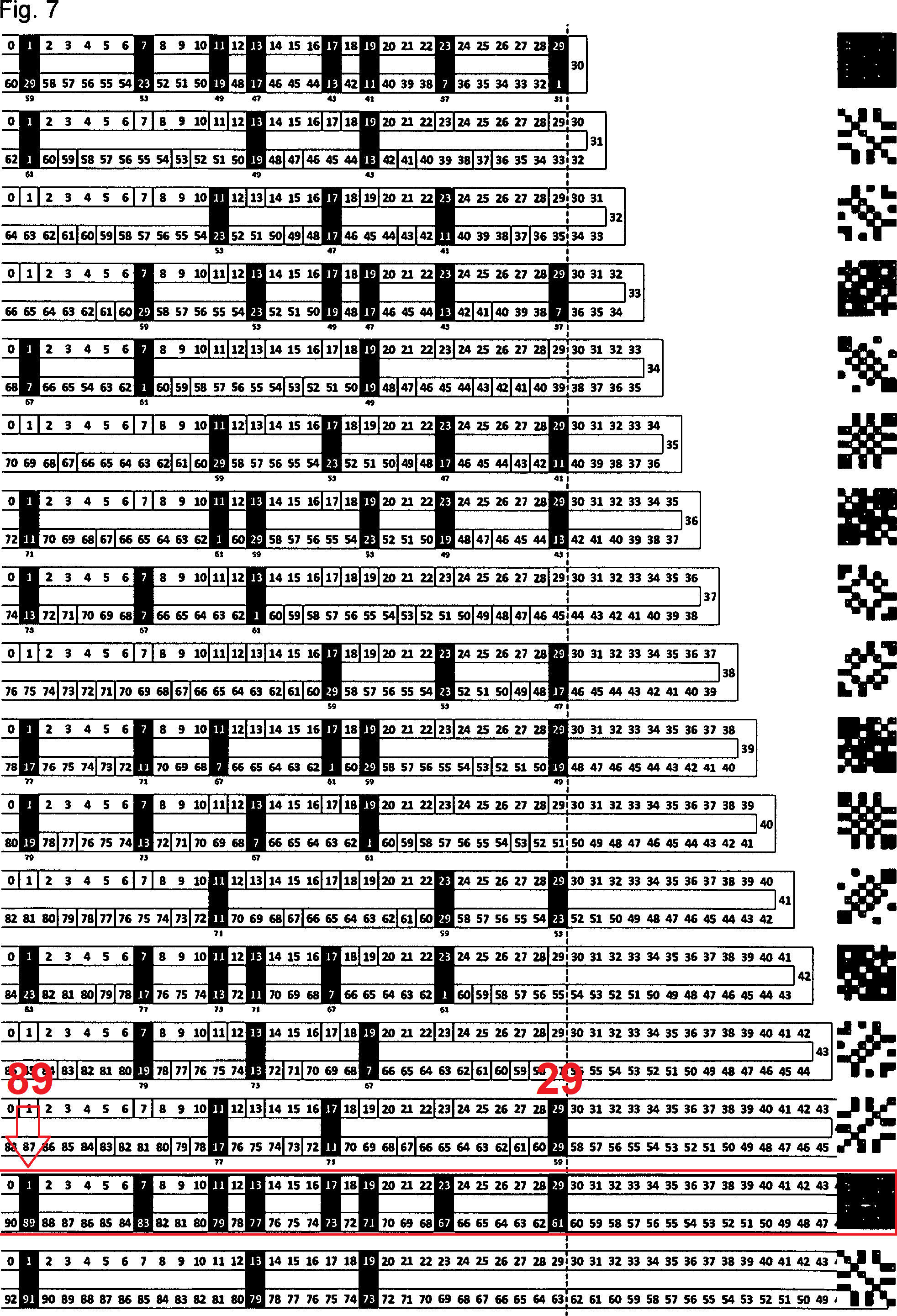## The Bilateral 9 Sums

Let's start with the number of possible ancestors on the chromosome. A male individual has an X chromosome, which he received from his mother, and a Y chromosome, which he received from his father.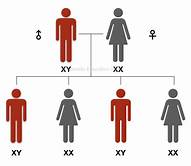The number of ancestors at each level, Fn, is the number of female ancestors, which is Fn−1, plus the number of male ancestors, which is Fn−2. his is under the unrealistic assumption that the ancestors at each level are otherwise unrelated.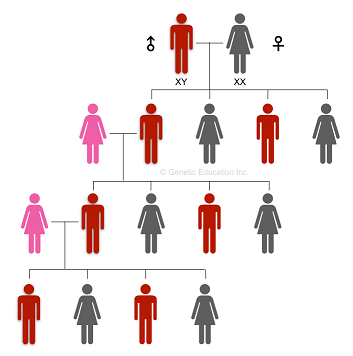It has been noticed that the number of possible ancestors on the human X chromosome inheritance line at a given ancestral generation also follows the Fibonacci sequence.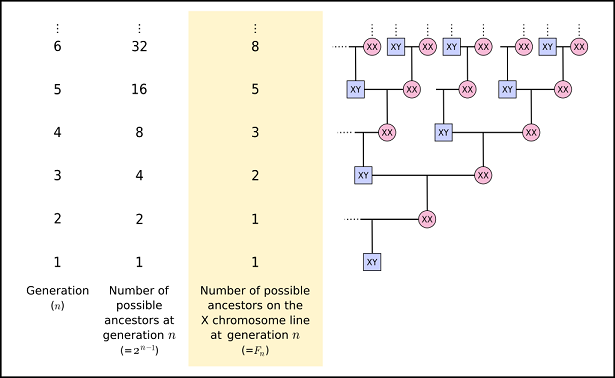This Fibonacci sequence is lied beautifully in the Pascal Triangle. Pascal's triangle has wide applications in Mathematics. We have seen that the most important applications relate to the binomial coefficients and combinatorics.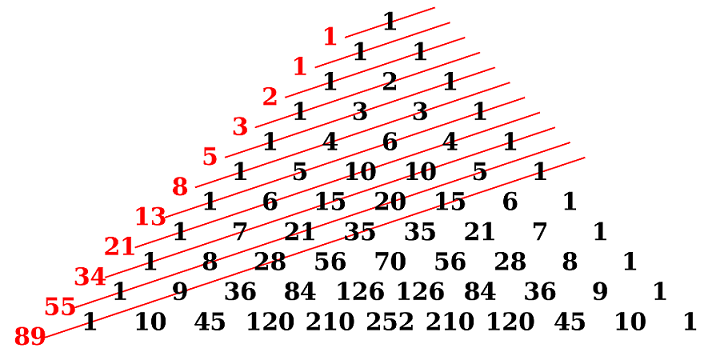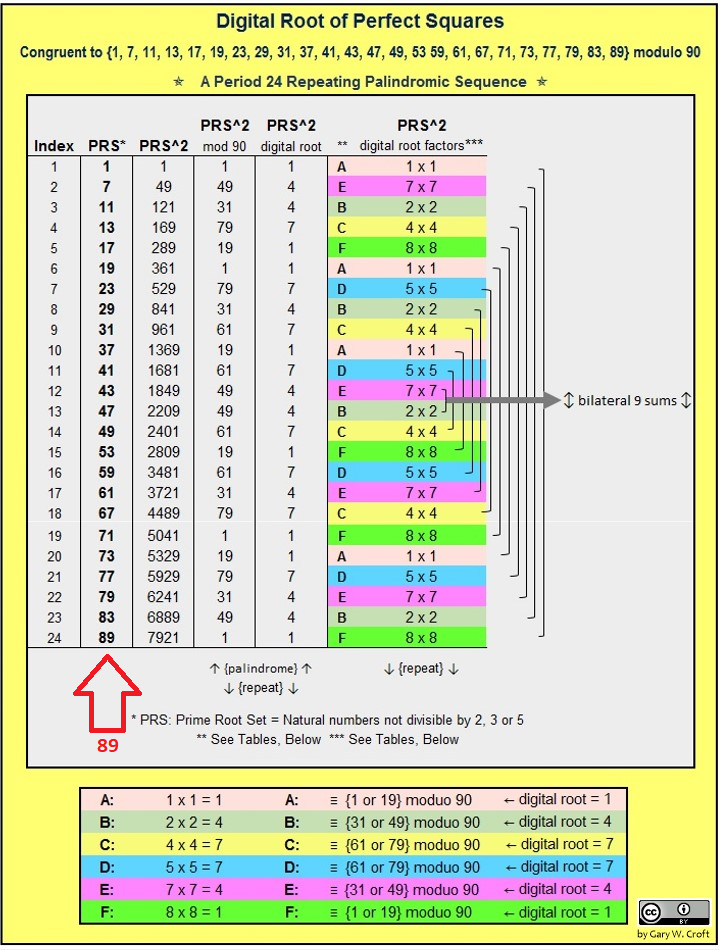See that from 1 to 89 it consist of seven (7) steps which is originated by 1,15,35,28 before it goes bilateral. So that is why Y has 1+15+35-1=50, and only 28-1=27 of these are in the male-specific. This cycle is repeated through a palindromic sequence.

109th - 20th = 89th

As the matter of fact this 89 has a symmetrical relation with the 24th Prime Number & 24th Natural Number Not Divisible by 2, 3, or 5. This is the reason that we use this primes pattern on determining the centralizing process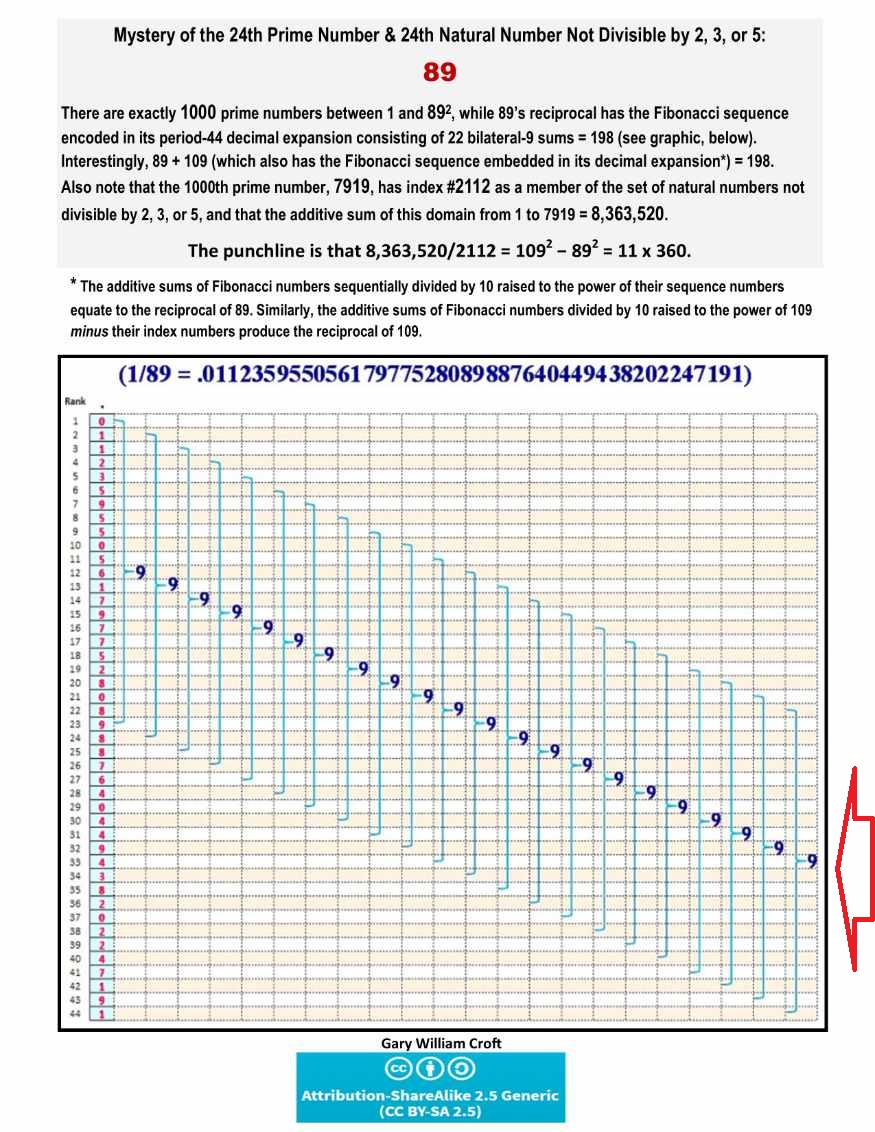So the 20 objects would pair another 19 and become 19 (sub objects) + 1 (unit object) + 19 (sub objects)=20+19=39 objects or it will be 40 when we put them as one (1) unit. This 40 will act as the interface between each of containers.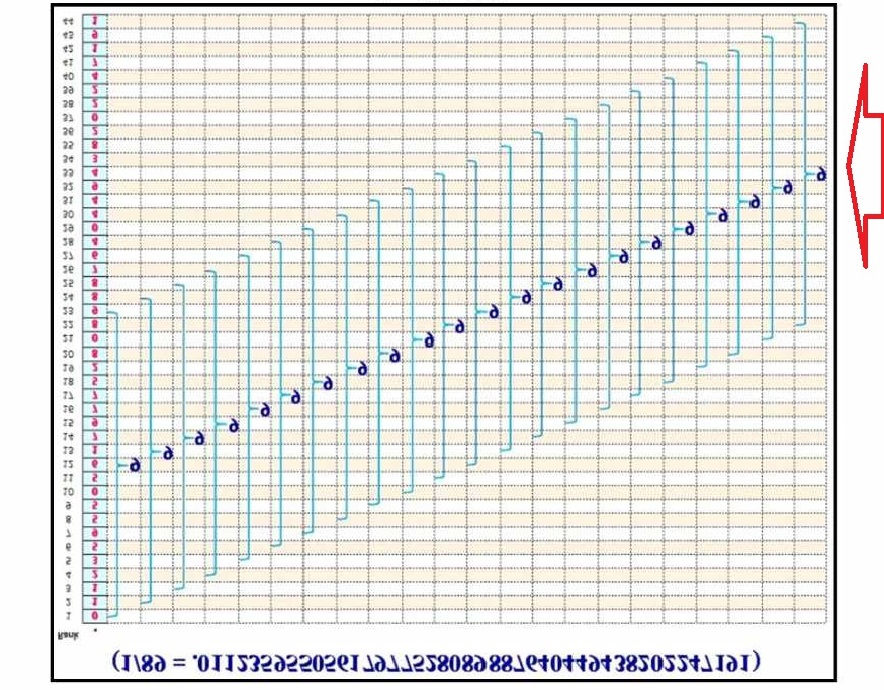This scheme stands as the second section of bilateral sum. By the rest sections we will discuss the above seven (7) steps one by one so all together will compromise the nine (9) groups of project repositories as mentioned in the beginning.